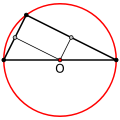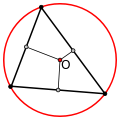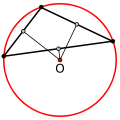## Circumcenter of Triangle Calculator

 Enter the coordinates of a triangle: X Y A: B: C: Triangle Circumcenter:

The circumcenter of a triangle is the perpendicular bisectors meet.

If you want to find the circumcenter of a triangle, First find the slopes and midpoints of the lines of triangle. Then perpendicular bisectors of the triangle lines, Last Solve any two pair of equations, The intersection point is the circumcenter.

For example, There points A (1, 3), B (5, 5), C (7, 5), the circumcenter is(6, -2).The circumcenter of a right triangle is at the center of the hypotenuseThe circumcenter of an acute triangle is inside the triangleThe circumcenter of an obtuse triangle is outside the triangle

Thinkcalculator.com provides you helpful and handy calculator resources.